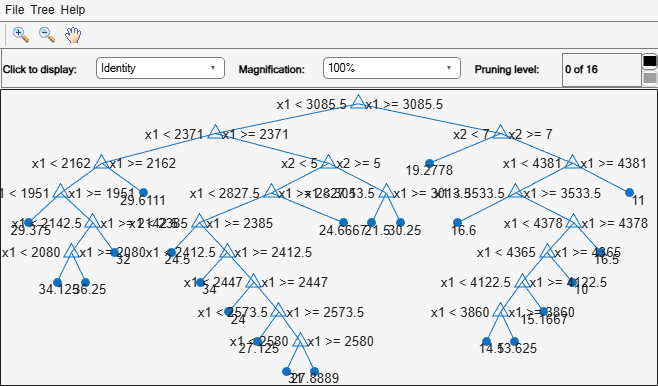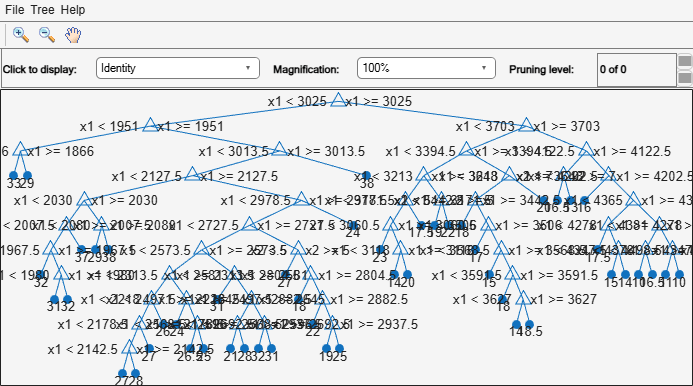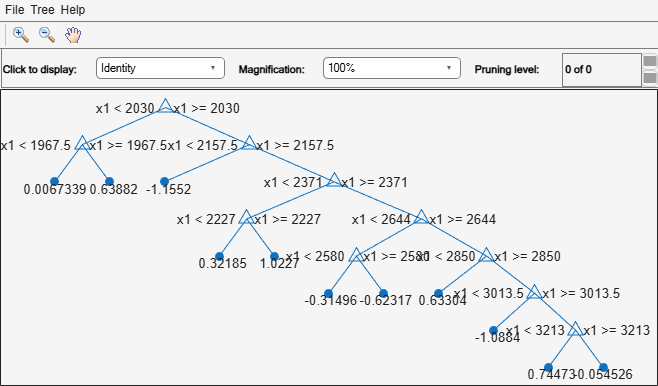# view

View regression tree

## Syntax

``view(tree)``
``view(tree,Mode=mode)``
``view(tree,"Mode",mode)``

## Description

example

````view(tree)` returns a text description of `tree`, a decision tree.```
````view(tree,Mode=mode)` also specifies the display mode as either graphical or text.Before R2021a, use the equivalent syntax `view(tree,"Mode",mode)`.```

## Examples

collapse all

View textual and graphical displays of a trained regression tree.

Load the `carsmall` data set. Consider a model that explains a car's fuel economy (`MPG`) using its weight (`Weight`) and number of cylinders (`Cylinders`).

```load carsmall X = [Weight Cylinders]; Y = MPG;```

Train a regression tree using all measurements.

`Mdl = fitrtree(X,Y);`

View textual display of the trained regression tree.

`view(Mdl)`
```Decision tree for regression 1 if x1<3085.5 then node 2 elseif x1>=3085.5 then node 3 else 23.7181 2 if x1<2371 then node 4 elseif x1>=2371 then node 5 else 28.7931 3 if x2<7 then node 6 elseif x2>=7 then node 7 else 15.5417 4 if x1<2162 then node 8 elseif x1>=2162 then node 9 else 32.0741 5 if x2<5 then node 10 elseif x2>=5 then node 11 else 25.9355 6 fit = 19.2778 7 if x1<4381 then node 12 elseif x1>=4381 then node 13 else 14.2963 8 if x1<1951 then node 14 elseif x1>=1951 then node 15 else 33.3056 9 fit = 29.6111 10 if x1<2827.5 then node 16 elseif x1>=2827.5 then node 17 else 27.2143 11 if x1<3013.5 then node 18 elseif x1>=3013.5 then node 19 else 23.25 12 if x1<3533.5 then node 20 elseif x1>=3533.5 then node 21 else 14.8696 13 fit = 11 14 fit = 29.375 15 if x1<2142.5 then node 22 elseif x1>=2142.5 then node 23 else 34.4286 16 if x1<2385 then node 24 elseif x1>=2385 then node 25 else 27.6389 17 fit = 24.6667 18 fit = 21.5 19 fit = 30.25 20 fit = 16.6 21 if x1<4378 then node 26 elseif x1>=4378 then node 27 else 14.3889 22 if x1<2080 then node 28 elseif x1>=2080 then node 29 else 34.8333 23 fit = 32 24 fit = 24.5 25 if x1<2412.5 then node 30 elseif x1>=2412.5 then node 31 else 28.0313 26 if x1<4365 then node 32 elseif x1>=4365 then node 33 else 14.2647 27 fit = 16.5 28 fit = 34.125 29 fit = 36.25 30 fit = 34 31 if x1<2447 then node 34 elseif x1>=2447 then node 35 else 27.6333 32 if x1<4122.5 then node 36 elseif x1>=4122.5 then node 37 else 14.5313 33 fit = 10 34 fit = 24 35 if x1<2573.5 then node 38 elseif x1>=2573.5 then node 39 else 27.8929 36 if x1<3860 then node 40 elseif x1>=3860 then node 41 else 14.15 37 fit = 15.1667 38 fit = 27.125 39 if x1<2580 then node 42 elseif x1>=2580 then node 43 else 28.2 40 fit = 14.5 41 fit = 13.625 42 fit = 31 43 fit = 27.8889 ```

View graphical display of the trained regression tree.

`view(Mdl,Mode="graph");`Load the `carsmall` data set. Consider a model that explains a car's fuel economy (`MPG`) using its weight (`Weight`) and number of cylinders (`Cylinders`).

```load carsmall X = [Weight Cylinders]; Y = MPG;```

Grow a bag of 100 regression trees using all measurements.

```rng(1) % For reproducibility Mdl = TreeBagger(100,X,Y);```

Alternatively, you can use `fitrensemble` to grow a bag of regression trees.

`Mdl` is a `TreeBagger` model object. `Mdl.Trees` stores the bag of 100 trained regression trees in a 100-by-1 cell array. That is, each cell in `Mdl.Trees` contains a `CompactRegressionTree` model object.

View a graph of the 10th regression tree in the bag.

```Tree10 = Mdl.Trees{10}; view(Tree10,'Mode','graph');```By default, the software grows deep trees for bags of trees.

Load the `carsmall` data set. Consider a model that explains a car's fuel economy (`MPG`) using its weight (`Weight`) and number of cylinders (`Cylinders`).

```load carsmall X = [Weight Cylinders]; Y = MPG;```

Boost an ensemble of 100 regression trees using all measurements.

`Mdl = fitrensemble(X,Y,'Method','LSBoost');`

`Mdl` is a `RegressionEnsemble` model object. `Mdl.Trained` stores the ensemble of 100 trained regression trees in a 100-by-1 cell array. That is, each cell in `Mdl.Trained` contains a `CompactRegressionTree` model object.

View a graph of the 10th regression tree in the ensemble.

```Tree10 = Mdl.Trained{10}; view(Tree10,'Mode','graph');```By default, `fitrensemble` grows shallow trees for boosted ensembles of trees. That is, `'Learners'` is `templateTree('MaxNumSplits',10)`.

## Input Arguments

collapse all

Regression tree, specified as a `RegressionTree` object created by the `fitrtree` function or a `CompactRegressionTree` object created by the `compact` function.

Display mode of `tree`, specified as `"graph"` or `"text"`. `"graph"` opens a GUI displaying `tree`, and containing controls for querying the tree. `"text"` sends output to the Command Window describing `tree`.

Data Types: `char` | `string`

## Tips

To view tree `t` from an ensemble of trees, enter one of these lines of code

```view(Ens.Trained{t}) view(Bag.Trees{t})```

To save `tree` in the Command Window, get a figure handle by using the `findall` and `setdiff` functions, and then save `tree` using the function `saveas`.

```before = findall(groot,'Type','figure'); % Find all figures view(Mdl,'Mode','graph') after = findall(groot,'Type','figure'); h = setdiff(after,before); % Get the figure handle of the tree viewer saveas(h,'a.png')```

## Version History

Introduced in R2011a